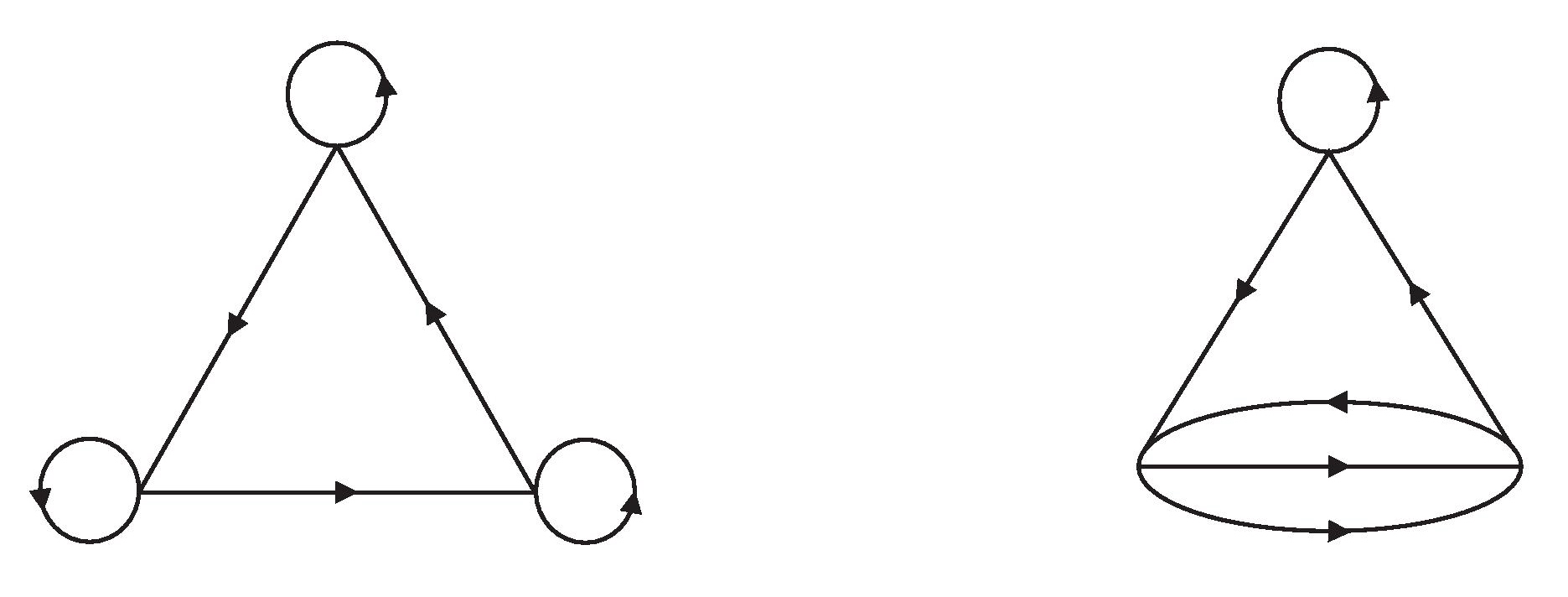# If $p$ is a regular covering then so is $q$.

If $$q:X\to Y$$, $$r:Y \to Z$$, and $$p=r \circ q : X \to Z$$ are all covering maps, with $$Z$$ locally path-connected, and if $$p$$ is a regular covering then so is $$q$$.

Note 1. The condition $$q$$ is a covering map is not necessary, because it is automatically satisfied.

Note 2. regular coverings are sometimes called normal coverings

Note 3.If $$X, Y, Z$$ are all path-connected, then this is easy, using the following fact:

Proposition. Let $$p : X \to Y$$ be a covering map with $$X$$ path-connected and $$Y$$ path-connected and locally path-connected. Then $$p$$ is a regular covering iff $$p_* \pi_1(X)$$ is a normal subgroup of $$\pi_1(Y)$$.

However, there are no assumptions that $$X, Y, Z$$ are path-connected. How do I have to proceed?

Let $$y \in Y$$ and $$x_1, x_2 \in q^{-1}(x)$$. We have to show that there is a deck transformation for $$q$$ taking $$x_1$$ to $$x_2$$.

Since $$x_1,x_2 \in p^{-1}(r(y))$$, we know that there exists a deck transformation $$d$$ for $$p$$ taking $$x_1$$ to $$x_2$$.

You assume that $$Z$$ locally path-connected. Hence also $$Y$$ and $$X$$ are locally path-connected. Thus all path-components of these spaces are open, and we conclude that the spaces are the disjoint union of their path-components. Let $$P_i$$ be the path-components of $$X$$ containing $$x_i$$. $$d$$ is a homeomorphism, hence it maps path components homeomorphically onto path components. Thus $$d(P_1) = P_2$$. You have either $$P_1 = P_2$$ or $$P_1 \cap P_2 = \emptyset$$. In the first case define a new deck transformation $$d'$$ for $$p$$ by $$d' \mid_{P_1} = d \mid_{P_1}$$ and $$d' \mid_P = id$$ for all path-components $$P \ne P_1$$. In the second case define a new deck transformation $$d'$$ for $$p$$ by $$d' \mid_{P_1} = d \mid_{P_1}$$, $$d' \mid_{P_2} = d^{-1} \mid_{P_2}$$ and $$d' \mid_P = id$$ for all path-components $$P \ne P_1, P_2$$. Then $$d'$$ is clearly a bijection. Both $$d', (d')^{-1}$$ are continuous (recall that $$X$$ is the disjoint union of its path components). By construction $$d'$$ takes $$x_1$$ to $$x_2$$.

We claim that $$d'$$ is deck transformation for $$q$$, that is $$q \circ d' = d'$$. Obviously $$(q \circ d')(x) = q(x)$$ for all $$x \notin P_1 \cup P_2$$. Let $$x \in P_1$$. There exists a path $$u$$ in $$P_1$$ such that $$u(0) = x_1$$ and $$u(1) = x$$. Consider the paths $$v' = q \circ d' \circ u$$ and $$v = q \circ u$$ in $$Y$$. They satisfy $$v'(0) = y = v(0)$$. We have $$r \circ v' = r \circ v$$, thus $$v' = v$$ by unique path lifting. Hence $$(q \circ d')(x) =(q \circ d' \circ u)(1) = (q \circ u)(1) = q(x)$$. Similarly we can show $$(q \circ d')(x) = q(x)$$ for $$x \in P_2$$. This proves $$q \circ d' = d'$$.

Remark.

We cannot expect that $$d$$ itself is deck transformation for $$q$$. As an example take $$r : Y = \{-1,1\} \to Z = \{0\}$$, $$q : X = \{-3,-2,-1,1,2,3\} \to Y, q(x) = \text{sgn}(x)$$. Here all spaces have the discrete topology. Let $$x_1 = 1, x_2 = 2$$. Then any permutation $$d$$ of $$X$$ taking such that $$d(1) = 2$$ is a deck transformation for $$p$$, but it is not a deck transformation for $$q$$ unless $$d(\{1,2,3\}) = \{1,2,3\}$$. Thus we must "adjust" $$d$$.

• Is $d'$ injective? What happens if $d(x_2) \notin P_1 \cup P_2$? Aug 17, 2019 at 1:42
• How about defining $d'=d$ on $P_1$, $d'=d^{-1}$ on $P_2$, and $d=$id otherwise (in the case $P_1 \neq P_2$) ? Aug 17, 2019 at 1:48
• Ah, you are right: There is no reason why $d(P_2) = P1$ if $P_1 \ne P_2$, thus we can have $d(P_1 \cup P_2) \ne P_1 \cup P_2$. The definition in your second comment will do. I corrected my answer. Thank you for drawing my attention to this point. Aug 17, 2019 at 8:39

The following is essentially 10.6.4 of Topology and Groupoids, using the "algebraic model" of covering maps of spaces by covering morphisms of greoupoids. Here a "loop" in a groupoid $$G$$ is an element of a vertex group $$G(x)= G(x,x)$$.

10.6.4 Let $$p : H \to G$$ be a covering morphism of groupoids. Consider the following conditions:

(a): for all loops $$a$$ in $$G$$, either all or no lifts of $$a$$ are loops;

(b): for all objects $$x$$ of $$H$$, the characteristic group $$p[H(x)]$$ is normal in $$G(px)$$.

Then (a} $$\Rightarrow$$ (b), and if $$H$$ is connected, (b) $$\Rightarrow$$ (a).

Fig 10.3 on the same page illustrates the idea.This should help to solve the problem easily, without mentioning paths.